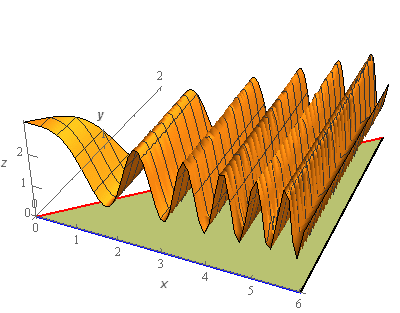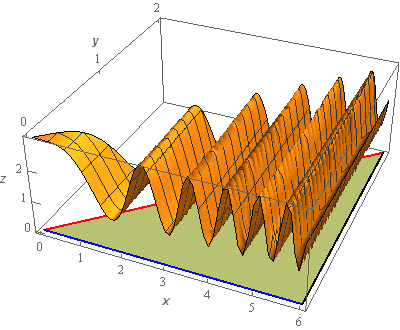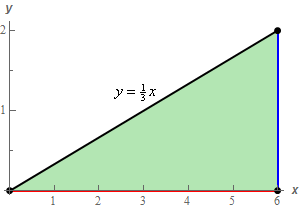Paul's Online Notes
Home / Calculus III / Multiple Integrals / Double Integrals over General Regions
Show Mobile Notice Show All Notes Hide All Notes
Mobile Notice
You appear to be on a device with a "narrow" screen width (i.e. you are probably on a mobile phone). Due to the nature of the mathematics on this site it is best views in landscape mode. If your device is not in landscape mode many of the equations will run off the side of your device (should be able to scroll to see them) and some of the menu items will be cut off due to the narrow screen width.

### Section 15.3 : Double Integrals over General Regions

13. Use a double integral to determine the volume of the region that is between the $$xy$$‑plane and$$f\left( {x,y} \right) = 2 + \cos \left( {{x^2}} \right)$$ and is above the triangle with vertices $$\left( {0,0} \right)$$, $$\left( {6,0} \right)$$ and $$\left( {6,2} \right)$$.

Show All Steps Hide All Steps

Start Solution

Let’s first get a sketch of the function and the triangle that lies under it.The surface is sketched with a traditional set of axes and well as a “box frame” set of axes. Sometimes it is easier to see what is going on with the surface when both sketches are present.

The greenish triangle underneath the surface is the triangle referenced in the problem statement.

Show Step 2

Now, the volume we are after is given by the following integral,

$V = \iint\limits_{D}{{2 + \cos \left( {{x^2}} \right)\,dA}}$

where $$D$$ is the triangle referenced in the problem statement.

So, in order to evaluate the integral we’ll need a sketch of $$D$$ so we can determine an order of integration as well as limits for the integrals.Show Step 3

The region $$D$$ can easily be described for either order of integration. However, it should be pretty clear that the integral can’t be integrated with respect to $$x$$ first and so we’ll need to integrate with respect to $$y$$ first.

Here are the limits for the integral with this order.

$\begin{array}{c} 0 \le x \le 6\\ 0 \le y \le \displaystyle \frac{1}{3}x\end{array}$

The integral for the volume is then,

$V = \iint\limits_{D}{{2 + \cos \left( {{x^2}} \right)\,dA}} = \int_{0}^{6}{{\int_{0}^{{\frac{1}{3}x}}{{2 + \cos \left( {{x^2}} \right)\,dy}}\,dx}}$ Show Step 4

Now all we need to do is evaluate the integral. Here is the $$y$$ integration.

$V = \int_{0}^{6}{{\left. {\left( {2y + y\cos \left( {{x^2}} \right)} \right)} \right|_0^{\frac{1}{3}x}\,dx}} = \int_{0}^{6}{{\frac{2}{3}x + \frac{1}{3}x\cos \left( {{x^2}} \right)\,dx}}$ Show Step 5

Finally, the $$x$$ integration and hence the volume is,

$V = \left. {\left( {\frac{1}{3}{x^2} + \frac{1}{6}\sin \left( {{x^2}} \right)\,} \right)} \right|_0^6 = \require{bbox} \bbox[2pt,border:1px solid black]{{12 + \frac{1}{6}\sin \left( {36} \right) = 11.8347}}$

Don’t forget to have your calculator set to radians if you are converting to decimals!